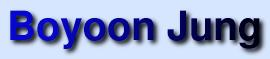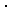Boyoon Jung's Library Manual

# QPosition Class Reference [Visualization Toolkit]

A Qt widget to display the poses of multiple sensors in 2D space. More...

`#include <qposition.h>`

List of all members.

## Public Slots

void setScale (double s)
Change the scaling factor.
void setTranslation (double tx, double ty)
Change the translation parameters.

## Signals

void scaleChanged (double)
Emitted when the scaling factor has been changed.
void translationChanged (double, double)
Emitted when the translation parameters have been changed.

## Public Member Functions

QPosition (QWidget *parent=0, const char *name=0, WFlags f=0)
A constructor.
int addSensor (const char *name, QColor color, Qt::PenStyle style)
Register a position sensor.
int addSensor (const char *name, QColor color)
Register a position sensor.
int addSensor (const char *name)
Register a position sensor.
bool setInitPose (double x, double y, double a=0, int id=0)
Set the initial position of a sensor.
bool setInitCovariance (double c00, double c01, double c10, double c11, int id=0)
Set the initial covariance of a sensor.
bool reset (double x=0, double y=0, double a=0, int id=0)
Reset the position of a sensor.
void add (double x, double y, double a=0, int id=0, bool flush=true)
Add a new position from a single sensor.
void add (double x, double y, double a, double c00, double c01, double c10, double c11, int id=0, bool flush=true)
Add a new position from a single sensor.
void add (double x, double y, double a, double c00, double c01, double c10, double c11, double c22, int id=0, bool flush=true)
Add a new position from a single sensor.
double scale (void) const
Return the scaling factor.
void translation (double &tx, double &ty)
Return the translation parameters.

## Protected Member Functions

void drawTrajectory (QPainter &p)
Draw trajectories.
void eigen (cov_t &m, double &w, double &h, double &a)
Compute the eigen values & vectors.
void mousePressEvent (QMouseEvent *e)
void mouseMoveEvent (QMouseEvent *e)
void mouseReleaseEvent (QMouseEvent *e)
void keyPressEvent (QKeyEvent *e)
void resizeEvent (QResizeEvent *e)
void drawContents (QPainter *p)
void drawContentsMask (QPainter *p)

## Detailed Description

A Qt widget to display the poses of multiple sensors in 2D space.

QPosition class provides a simple way to visualize the trajectories of multiple sensors (i.g. a robot odometer or a GPS) moving in 2D space. The unit of position is meter, and the unit of heading is radian.

## Constructor & Destructor Documentation

 QPosition::QPosition ( QWidget * parent = `0`, const char * name = `0`, WFlags f = `0` )
 A constructor.

## Member Function Documentation

 void QPosition::add ( double x, double y, double a, double c00, double c01, double c10, double c11, double c22, int id = `0`, bool flush = `true` )

Add a new position from a single sensor.

Parameters:
 x The X position in meter. y The X position in meter. a The heading in radian. c00 The element at (0,0) of the covariance matrix. c01 The element at (0,1) of the covariance matrix. c10 The element at (1,0) of the covariance matrix. c11 The element at (1,1) of the covariance matrix. c22 The element at (2,2) of the covariance matrix. id The sensor ID. flush Re-draw tracks immediately.

 void QPosition::add ( double x, double y, double a, double c00, double c01, double c10, double c11, int id = `0`, bool flush = `true` )

Add a new position from a single sensor.

Parameters:
 x The X position in meter. y The X position in meter. a The heading in radian. c00 The element at (0,0) of the covariance matrix. c01 The element at (0,1) of the covariance matrix. c10 The element at (1,0) of the covariance matrix. c11 The element at (1,1) of the covariance matrix. id The sensor ID. flush Re-draw tracks immediately.

 void QPosition::add ( double x, double y, double a = `0`, int id = `0`, bool flush = `true` )

Add a new position from a single sensor.

Parameters:
 x The X position in meter. y The X position in meter. a The heading in radian. id The sensor ID. flush Re-draw tracks immediately.

 int QPosition::addSensor ( const char * name ) ` [inline]`
 Register a position sensor.

 int QPosition::addSensor ( const char * name, QColor color ) ` [inline]`
 Register a position sensor.

 int QPosition::addSensor ( const char * name, QColor color, Qt::PenStyle style )
 Register a position sensor.

 void QPosition::drawContents ( QPainter * p ) ` [protected]`

 void QPosition::drawContentsMask ( QPainter * p ) ` [inline, protected]`

 void QPosition::drawTrajectory ( QPainter & p ) ` [protected]`
 Draw trajectories.

 void QPosition::eigen ( cov_t & m, double & w, double & h, double & a ) ` [protected]`
 Compute the eigen values & vectors.

 void QPosition::keyPressEvent ( QKeyEvent * e ) ` [protected]`

 void QPosition::mouseMoveEvent ( QMouseEvent * e ) ` [protected]`

 void QPosition::mousePressEvent ( QMouseEvent * e ) ` [protected]`

 void QPosition::mouseReleaseEvent ( QMouseEvent * e ) ` [protected]`

 bool QPosition::reset ( double x = `0`, double y = `0`, double a = `0`, int id = `0` )
 Reset the position of a sensor.

 void QPosition::resizeEvent ( QResizeEvent * e ) ` [inline, protected]`

 double QPosition::scale ( void ) const` [inline]`
 Return the scaling factor.

 void QPosition::scaleChanged ( double ) ` [signal]`
 Emitted when the scaling factor has been changed.

 bool QPosition::setInitCovariance ( double c00, double c01, double c10, double c11, int id = `0` )
 Set the initial covariance of a sensor.

 bool QPosition::setInitPose ( double x, double y, double a = `0`, int id = `0` )
 Set the initial position of a sensor.

 void QPosition::setScale ( double s ) ` [inline, slot]`
 Change the scaling factor.

 void QPosition::setTranslation ( double tx, double ty ) ` [inline, slot]`
 Change the translation parameters.

 void QPosition::translation ( double & tx, double & ty ) ` [inline]`
 Return the translation parameters.

 void QPosition::translationChanged ( double , double ) ` [signal]`
 Emitted when the translation parameters have been changed.

The documentation for this class was generated from the following file:
ResearchProjectsPublications

ToolsBjLib ManualPlayer/StagePyPlayerMicroStrain IMUGPS/DGPSParticle Filter

PersonalWelcomeCurriculum VitaeResumeContact

Outside LinksUSCUSC CS DeptCRESRobotics LabRESL